Thermodynamics and Propulsion
Subsections

# 19.3 Radiation Heat Transfer Between Planar Surfaces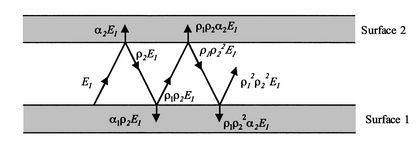Consider the two infinite gray surfaces shown in Figure 19.5. We suppose that the surfaces are thick enough so that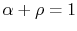(no radiation transmitted so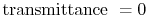). Consider a photon emitted from Surface 1 (remembering that the reflectance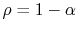):

 Surface 1 emitsSurface 2 absorbsSurface 2 reflectsSurface 1 absorbs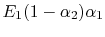Surface 1 reflects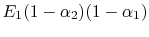Surface 2 absorbsSurface 2 reflects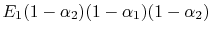Surface 1 absorbs...

The same can be said for a photon emitted from Surface 2:

 Surface 2 emits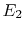Surface 1 absorbsSurface 1 reflects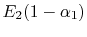Surface 2 absorbsSurface 2 reflects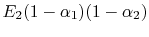...

We can add up all the energyabsorbed in 1 and all the energyabsorbed in 2. In doing the bookkeeping, it is helpful to define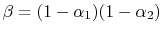. The energyabsorbed in 1 isThis is equal toHowever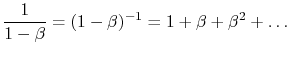We thus observe that the radiation absorbed by surface 1 can be written asLikewiseis the radiation generated at 2 and absorbed there as well. Putting this all together we find thatis absorbed by 1. The net heat flux from 1 to 2 is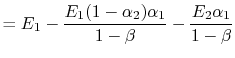or(19..2)

If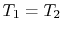, we would have, so from Equation 19.2,If body 2 is black,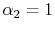, and.Therefore, again,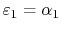for any gray surface (Kirchhoff's Law).

Using Kirchhoff's Law we find,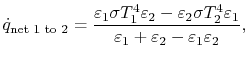or, as the final expression for heat transfer between gray, planar, surfaces,(19..3)

## 19.3.1 Example 1: Use of a thermos bottle to reduce heat transferfor silvered walls.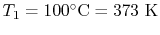;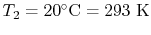.For the same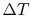, if we had cork insulation with, what thickness would be needed?so a thickness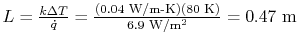would be needed! The thermos is indeed a good insulator.

## 19.3.2 Example 2: Temperature measurement error due to radiation heat transferThermocouples (see Figure 19.7) are commonly used to measure temperature. There can be errors due to heat transfer by radiation. Consider a black thermocouple in a chamber with black walls.

Suppose the air is at, the walls are at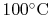, and the convective heat transfer coefficient is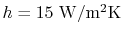.

What temperature does the thermocouple read?We use a heat (energy) balance on the control surface shown in Figure 19.8. The heat balance states that heat convected away is equal to heat radiated into the thermocouple in steady state. (Conduction heat transfer along the thermocouple wires is neglected here, although it would be included for accurate measurements.)

The heat balance is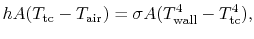where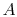is the area of the thermocouple. Substituting the numerical values gives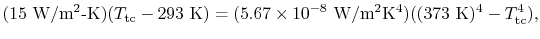from which we find. The thermocouple thus sees a higher temperature than the air. We could reduce this error by shielding the thermocouple as shown in Figure 19.9.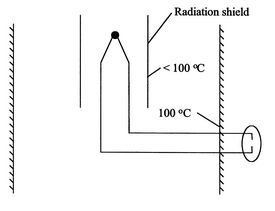Muddy Points

Which bodies does the radiation heat transfer occur between in the thermocouple?(MP 19.1)

Still muddy about thermocouples. (MP 19.2)

Why does increasing the local flow velocity decrease the temperature error for the thermocouple? (MP 19.3)

UnifiedTP